Short Answer Type Questions- Areas of Parallelograms and Triangles

# Short Answer Type Questions- Areas of Parallelograms and Triangles - Mathematics (Maths) Class 9

Q1. PQRS is a square. T and U are respectively, the mid points of PS and QR. Find the area of ΔOTS, if PQ = 8 cm, where O is the point of intersection of TU and OS.

We have, PS = PQ = 8 cm and TU || PQ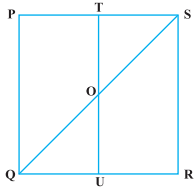∵ ST = (1/2) PS
∴ ST = (1/2)× 8 = 4 cm
Also, PQ = TU = 8 cm
∴ OT = (1/2)TU = (1/2) × 8 = 4 cm
Now, Area of ΔOTS
= (1/2)× ST × OT           [∵ OTS is a rt. triangle]
= (1/2)× 4 × 4 cm2
= 8 cm2

Q2. The area of the parallelogram PQRS (in the adjoining figure) is 88 cm2. Find the value of x.

PQRS is a parallelogram.
Area of a parallelogram = base x height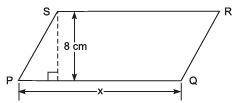x * 8 cm = 88 cm2
⇒ x= (88/8) cm
⇒ x= 11 cm Thus, the required value of x is 11 cm.

Q3. Find the area of ΔABC given in the adjoining figure.

∵The area of a triangle = (1/2)x base x altitude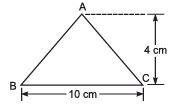∴ Area of ΔABC = (1/2)x 10 cm x 4 cm
= 20 cm2

Q4. In the adjoining figure, ABCD is a parallelogram and BPC is a triangle. If the area of parallelogram ABCD = 26 cm2, then find the area of triangle BPC.

Since parallelogram ABCD and ΔBPC are on the same base BC and between the same parallels BC and AD.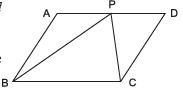∴ Area of ΔBPC = (1/2) x Area of parallelogram ABCD
⇒ Area of ΔBPC =(1/2) x  26 cm
= 13 cm2
∴ The required area of ΔBPC = 13 cm2.

Q5. In the adjoining figure, PQRS is a parallelogram and PQT is a triangle. If area of triangle PQT = 18 cm2, then find the area of the parallelogram PQRS.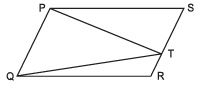∵ Parallelogram PQRS and ΔPQT are on the same base PQ and between the same parallels PQ and SR.
∴ Area of parallelogram PQRS = 2(ar ΔPQT)
⇒ ar (parallelogram PQRS) = 2(18 cm2)
⇒ ar (parallelogram PQRS)
= 36 cm2
Thus, the required area of parallelogram PQRS is 36 cm2.

Q6. In the adjoining figure. ABC is triangle and AD is a median. If the area of ΔABD is 15 cm2, then find the area of ΔABC.

Since, a median divides the triangle into two triangles of equal areas.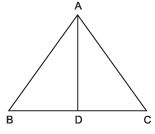∴ ar ΔABD = (1/2)(ar ΔABC)
⇒ 2(ar ΔABD) = ar (ΔABC)
⇒ 2(15 cm2) = ar (ΔABC)
⇒ 30 cm2 = ar (ΔABC)
Thus, the required area of ΔABC is 30 cm2.

Q7. The area of ΔABC, in the adjoining figure, is 32 cm2. AD is a median and E is the mid-point of AD. Find the area of ΔBED.

Since, the median of a triangle, divides it into triangles of equal areas.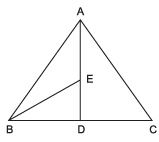∴ ar (ΔABD) =(1/2)x ar (ΔABC)              ...(1)
Similarly, ar (ΔBED) = (1/2) x ar (ΔABD)                      ...(2)
From (1) and (2), we have ar (ΔBED)
= (1/2)x (1/2)x [ar (ΔABC)]
= (1/4) ar (ΔABC)
= (1/4)x 32 cm= 8 cm2
Thus, the area of ΔBED is 8 cm2.

Q8. In the adjoining figure, the area of ΔBCE is 21 cm2. If CD = 6 cm, then find the length of AF.

∵ Parallelogarm ABCD and ΔBCE are on the same base and between the same parallels.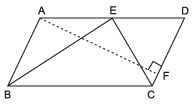∴ ar (ΔBCE) = (1/2) x  ar (parallelogram ABCD)
⇒ 21 cm2 = (1/2)x ar (parallelogram ABCD)
⇒ 21 cm2 = (1/2) [CD x AF]
⇒ 21 cm2 = (1/2)[6 x AF]
⇒ AF = ((21 x 2)/2) cm = 7 cm
Thus, the required length of AF is 7 cm.

Q9. ABCD is a parallelogram and BC is produced to a point Q such that AD = CQ. If AQ intersects DC at P, show that: ar (BPC) = ar (DPQ)

∵  The triangles on the same base and between same parallels.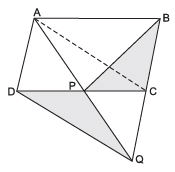∴ ar (ΔACP) = ar (ΔBPC)             .. (1)
⇒ ar (ΔAPC) = ar (ΔAPQ)                    ... (3)
From (1) and (3), we get
ar (ΔBCP) = ar (ΔDPQ)

Q10. In the adjoining figure, the area of a parallelogram ABCD is 40 cm2. If PQ is a median of ΔCDP then, find the area of ΔPDQ.

ar (ΔCDP) = (1/2) (Area of parallelogram ABCD)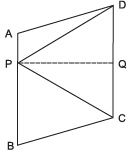= (1/2) (40 cm2) = 20 cm
∵ PQ is a median of ΔCDP.
∴ ar (ΔPDQ) = (1/2) x ar (ΔCDP)
⇒ ar (ΔPDQ) = (1/2) x (20 cm2) = 10 cm
Thus, the required area of ΔPDQ is 10 cm2.

Q11. In the adjoining figure, ABC is a triangle having area as 24 cm2. Find the area of (i) ΔEFD and (ii) parallelogram BDEF such that E, F and D are the mid-points of sides CA, AB and BC respectively.

Since, D, E and F are the mid-points of sides BC, CA and AB respectively.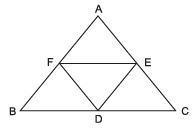∴ ar (ΔDEF) = (1/4) (ar ΔABC)
ar (ΔDEF) = (1/4)(24 cm2) = 6 cm2
Thus, the required area of ΔDEF = 6 cm2.
ar (parallelogram BDEF) = ar (ΔBDF) + ar (ΔDEF)
= (1/4)x ar (ΔABC) + (1/4)x ar (ΔABC)
= (1/4)x (24 cm2) +(1/4) x (24 cm2)
= 6 cm+ 6 cm2
= 12 cm2
Thus, the required area of parallelogram BDEF = 12 cm2.

The document Short Answer Type Questions- Areas of Parallelograms and Triangles | Mathematics (Maths) Class 9 is a part of the Class 9 Course Mathematics (Maths) Class 9.
All you need of Class 9 at this link: Class 9

## FAQs on Short Answer Type Questions- Areas of Parallelograms and Triangles | Mathematics (Maths) Class 9 PDF Download

 1. What is the formula to calculate the area of a parallelogram?Ans. The formula to calculate the area of a parallelogram is base multiplied by the height.
 2. How can I find the height of a parallelogram if the area and base are given?Ans. To find the height of a parallelogram, divide the area by the base.
 3. What is the formula to calculate the area of a triangle?Ans. The formula to calculate the area of a triangle is 1/2 multiplied by base multiplied by height.
 4. Can the area of a parallelogram be negative?Ans. No, the area of a parallelogram cannot be negative. Area is a measure of space and cannot have a negative value.
 5. How is the area of a triangle related to the area of a parallelogram?Ans. The area of a triangle is half the area of a parallelogram. This is because a parallelogram can be divided into two congruent triangles.

## Mathematics (Maths) Class 9

62 videos|426 docs|102 tests

## Mathematics (Maths) Class 9

62 videos|426 docs|102 tests
Signup to see your scores go up within 7 days! Learn & Practice with 1000+ FREE Notes, Videos & Tests.
10M+ students study on EduRev
Track your progress, build streaks, highlight & save important lessons and more!(Scan QR code)
Related Searches

,

,

,

,

,

,

,

,

,

,

,

,

,

,

,

,

,

,

,

,

,

;